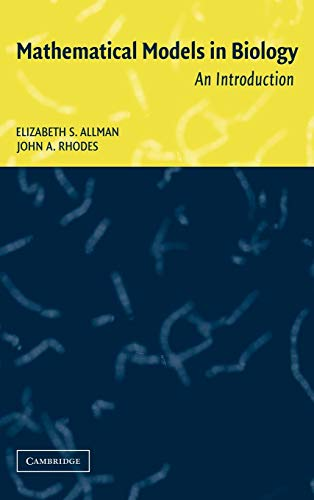## Mathematical models in biology : an introduction

Algunos libros útiles de matemáticas difíciles de conseguir.
Leonardo Sáenz
Mensajes: 2850
Registrado: Mié Jul 02, 2008 11:58 am
Verificación antispam: 4
Contactar:

### Mathematical models in biology : an introductionMathematical models in biology : an introduction, Elizabeth Spencer Allman; John A Rhodes
Focusing on discrete models across a variety of biological subdisciplines, this introductory textbook includes linear and non-linear models of populations, Markov models of molecular evolution, phylogenetic tree construction from DNA sequence data, genetics, and infectious disease models. Assuming no knowledge of calculus, the development of mathematical topics, such as matrix algebra and basic probability, is motivated by the biological models. Computer research with MATLAB is incorporated throughout in exercises and more extensive projects to provide readers with actual experience with the mathematical models.